# Automatically Tune Tracking Filter for Multi-Object Tracker

This example shows how to automatically tune a tracking filter using the `trackingFilterTuner` object. After tuning, use the tuning results in a multi-target tracker to improve the tracking performance of the tracker.

### Run a Tracker with an Untuned Filter

This example builds upon the scenario from the Air Traffic Control example.

To study whether filter tuning is required, construct a `trackerGNN` System object with the default filter initialization function. Additionally, define an optimal sub-pattern association (OSPA) metric, `trackOSPAMetric`, to quantitatively analyze the tracking results. As a reminder, lower OSPA values mean better tracking quality. Use the slider to adjust the assignment threshold.

```tracker = trackerGNN(AssignmentThreshold =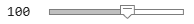100); metric = trackOSPAMetric(Distance = "posabserr", CutoffDistance = 200);```

Compared with the original example, the radar is modified to have wider beam and a faster radar scanning speed, which allows it to generate more detections of each target in the scenario.

```load("ATCScenario.mat","scenario"); helperRunScenario(scenario, tracker, metric);```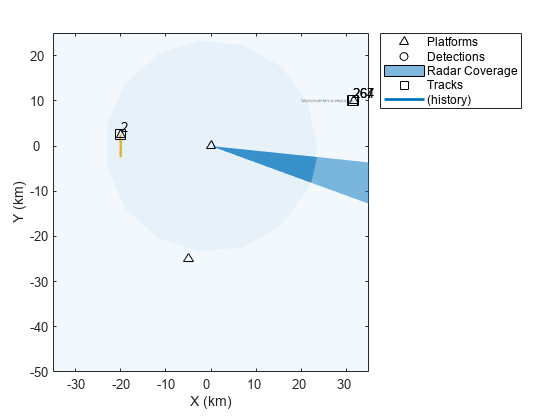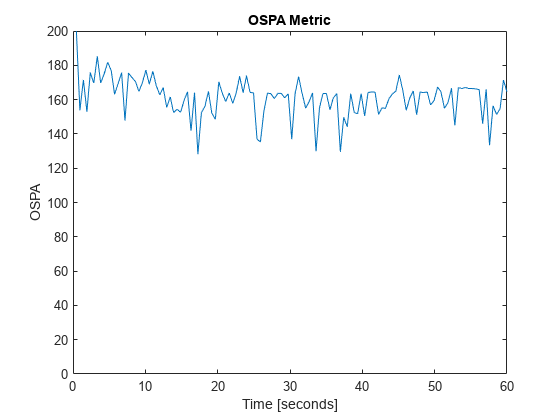From the results. even when the assignment threshold is set at the highest, the tracker performs undesirably and is unable to track all the targets.

### Create Tuning Data from the Scenario

To automatically tune a filter, use the `trackingFilterTuner` object with the history of truth objects and a log of detections per each truth object. The truth data is a cell array of `timetable` objects with each cell representing the history of one truth object. The detection log is a cell array. The $\mathit{i}$-th cell in the detection log is a cell array of `objectDetectionDelay` objects corresponding to the $\mathit{i}$-th truth object in the truth data.

To create the tuning data from the scenario, use the `monteCarloRun` function, which creates scenario recordings with different noise realizations. To convert the recordings to tuning data, use the `helperRecordingToTuningData` supporting function attached in the example. For brevity, this example directly loads a file with tuning data, created by two Monte Carlo runs of the scenario.

```% recording = monteCarloRun(scenario,2); % [detections, truthTable] = recordingToTuningData(recording); load("ATCTuningData.mat","detections","truthTable"); helperVisualizeTuningData(detections,truthTable);```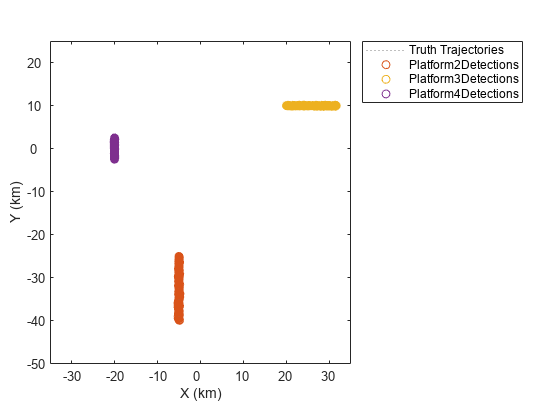### Tune a Filter with Default Tuning Parameters

To understand the difficulty of the tracker in tracking the fast-moving platforms, look at the filter that is initialized by the default `initcvekf` function used in the tracker.

```sampleDetection = detections{1}{1}; filter = initcvekf(sampleDetection);```

The filter uses the constant velocity motion model convention, in which the state vector defined as: $\stackrel{\to }{\text{\hspace{0.17em}}\mathit{x}}={\left[\mathit{x},{\mathit{v}}_{\mathit{x}},\mathit{y},{\mathit{v}}_{\mathit{y}},\text{\hspace{0.17em}}\mathit{z},{\mathit{v}}_{\mathit{z}}\right]}^{\mathit{T}}$, where $\mathit{x}$, $\mathit{y}$, and $\mathit{z}$ are position components in the rectangular frame and ${\mathit{v}}_{\mathit{x}}$, ${\mathit{v}}_{\mathit{y}}$, and ${\mathit{v}}_{\mathit{z}}$ are the velocity components. The process noise of the filter represents the uncertainty about the velocity change, or acceleration, of the object.

`disp(filter.ProcessNoise);`
``` 1 0 0 0 1 0 0 0 1 ```

Similarly, the state covariance represents the uncertainty in the position and velocity components. The `initcvekf` function directly uses the detection measurement noise to initialize the position components in the state uncertainty covariance. However, since the velocity components are not reported in the measurement, their respective uncertainty is set to 100 by default, representing a standard deviation of 10 m/s. Obviously, for airliners, the uncertainty should be higher.

`disp(filter.StateCovariance);`
``` 1.0e+04 * 0.9582 0 -0.1154 0 -0.0824 0 0 0.0100 0 0 0 0 -0.1154 0 0.0488 0 -0.3422 0 0 0 0 0.0100 0 0 -0.0824 0 -0.3422 0 4.1085 0 0 0 0 0 0 0.0100 ```

Create a `trackingFilterTuner` object.

```tuner = trackingFilterTuner; disp(tuner)```
``` trackingFilterTuner with properties: FilterInitializationFcn: "initcvekf" TunablePropertiesSource: "Default" Cost: "RMSE" UseMex: 0 UseParallel: 0 Solver: "active-set" Display: "Text" ```

By default, when tuning a `trackingEKF` object that is initialized by the `initcvekf` function, the tuner tunes the `ProcessNoise` property of the filter and uses the root mean square error (RMSE) between the filter estimate and the truth as the cost. The process noise is usually the hardest to define, because often there is little information about how the object motion differs from the motion model used in the filter. The initial state covariance is the next hardest thing to tune, which you will tune in the next section.

To accelerate the tuning, set the `UseMex` property to `true` using the checkbox. This setting requires a MATLAB Coder license. Uncheck the box if that license is not available.

`tuner.UseMex =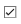true;`

Similarly, set the `UseMex` property to true to use parallel processing, which requires a Parallel Computing Toolbox license. Uncheck the box if that license is unavailable.

`tuner.UseParallel =true;`

You can use one of three solvers based on the `fmincon`, `particleswarm`, and `patternsearch` optimization algorithms. Choose your optimization algorithm based on the complexity of the optimization problem, the time allowed for it to run, and other criteria. You can refer to the Optimization Toolbox™ and Global Optimization Toolbox™ documentation to decide which solver to use.

The `'fmincon'` solver requires the Optimization Toolbox license.

The `'patricleswarm'` or the `'patternsearch'` solvers require the Global Optimization Toolbox license.

`solver =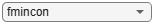"fmincon";`

Set the solver options to provide an iterative display of the solving progress over time. Use the slider to control the maximum number of iterations the solver can use. Setting the number of iterations higher requires more time for the tuner. To tune a filter to track all the targets, use the tuning data for all the targets.

```tuner.Solver = solver; tuner.SolverOptions = optimoptions(solver, Display = "iter", ... MaxIter =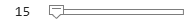15); tic; [tunedParams, bestCost] = tune(tuner,detections,truthTable);```
```Starting parallel pool (parpool) using the 'Processes' profile ... Connected to parallel pool with 6 workers. Generating code. Code generation successful. Your initial point x0 is not between bounds lb and ub; FMINCON shifted x0 to strictly satisfy the bounds. Iter RMSE Step Size 0 78.4801 0.0000 First-order Norm of Iter F-count f(x) Feasibility optimality step 0 7 7.848006e+01 0.000e+00 1.613e-01 1 14 7.839574e+01 0.000e+00 1.852e-01 3.538e-01 1 78.3957 0.3538 2 21 7.810211e+01 0.000e+00 1.639e-01 1.463e+00 2 78.1021 1.4628 3 28 7.768567e+01 0.000e+00 1.813e-01 3.044e+00 3 77.6857 3.0440 4 35 7.720462e+01 0.000e+00 2.038e-01 4.543e+00 4 77.2046 4.5434 5 43 7.703294e+01 0.000e+00 2.229e-01 1.866e+00 5 77.0329 1.8661 6 50 7.686042e+01 0.000e+00 2.013e-01 1.277e+00 6 76.8604 1.2772 7 57 7.654589e+01 0.000e+00 1.457e-01 1.737e+00 7 76.5459 1.7370 8 64 7.603169e+01 0.000e+00 6.552e-02 4.124e+00 8 76.0317 4.1242 9 71 7.600035e+01 0.000e+00 6.463e-02 8.611e-01 9 76.0003 0.8611 10 78 7.589348e+01 0.000e+00 6.237e-02 1.662e+00 10 75.8935 1.6618 11 85 7.592252e+01 0.000e+00 4.813e-02 5.195e-01 11 75.9225 0.5195 12 92 7.591004e+01 0.000e+00 4.924e-02 1.346e-01 12 75.9100 0.1346 13 99 7.588873e+01 0.000e+00 3.311e-02 3.816e-01 13 75.8887 0.3816 14 106 7.586347e+01 0.000e+00 3.171e-02 1.262e+00 14 75.8635 1.2622 15 113 7.584819e+01 0.000e+00 2.707e-02 1.731e+00 15 75.8482 1.7310 Solver stopped prematurely. fmincon stopped because it exceeded the iteration limit, options.MaxIterations = 1.500000e+01. ```
`disp("Time it took to tune the filter: " + toc);`
```Time it took to tune the filter: 135.5945 ```
`disp("Optimized cost: " + bestCost);`
```Optimized cost: 75.8482 ```

Note that the optimization process has not improved the cost much. That is expected, because in this case the main driver of the high cost is the poor initial velocity covariance, which was not part of the tuning.

The tuned properties for the `trackingEKF` object are returned as a structure with two fields: `ProcessNoise` and `StateCovariance`. Their values are displayed below. The state covariance is not tuned.

`disp(tunedParams.ProcessNoise);`
``` 111.6332 28.3525 96.7912 28.3525 48.7698 13.3867 96.7912 13.3867 97.0984 ```
`disp(tunedParams.StateCovariance);`
``` 1.0e+04 * 0.9582 0 -0.1154 0 -0.0824 0 0 0.0100 0 0 0 0 -0.1154 0 0.0488 0 -0.3422 0 0 0 0 0.0100 0 0 -0.0824 0 -0.3422 0 4.1085 0 0 0 0 0 0 0.0100 ```

To obtain the tuned filter, either set each property on the filter to its corresponding tuned value from the structure or use `setTunedProperties` object function of the filter.

```filter = feval(tuner.FilterInitializationFcn, sampleDetection); setTunedProperties(filter,tunedParams); disp(filter.ProcessNoise);```
``` 111.6332 28.3525 96.7912 28.3525 48.7698 13.3867 96.7912 13.3867 97.0984 ```

Use the `plotFilterErrors` object function to show the estimate accuracy. The plots show the estimation errors of the position and the velocity in all motion dimensions. Each line represents the error between the estimate from one run of the filter and the associated truth. The bands represent the three standard deviations ("3-sigma") in that dimension obtained from the filter state covariance. For an unbiased filter, the filter estimate error should average around zero.

Furthermore, the estimate of a consistent Gaussian filter should fall within the 3-sigma bounds about 99% of the time. If the filter is overconfident, there will be more violations of the three-standard deviations bounds. If the filter is underconfident, the actual filter errors will be very small relative to the bounds. Having a consistent filter that provides the right measure of uncertainty is important in tracking.

`plotFilterErrors(tuner);`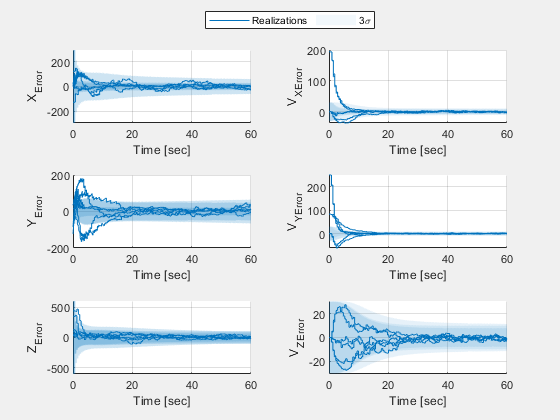### Customize the Tuning

When tuning only the process noise, the filter estimate errors, especially for the velocity along the X and Y axes, far exceeds the three standard deviation bounds. The reason is that the initial state covariance value for velocity is too low. In other words, the filter is too confident about its velocity estimate. It also causes the process noise value to be too high after tuning, because the tuning algorithms tries to compensate for the large initial errors. As a result, the estimate follows the measurement more closely than it should and the filter error is large even after the filter converges.

To fix this issue, set the tuner to use custom properties. To create the filter tunable properties, create a same type of tracking filter object with the same motion model used in the tracking. Next, use the `tunableProperties` object function of the filter to construct a `tunableFilterProperties` object.

```filter = feval(tuner.FilterInitializationFcn, sampleDetection); tfp = tunableProperties(filter); disp(tfp)```
```Tunable properties for object of type: trackingEKF Property: ProcessNoise PropertyValue: [1 0 0;0 1 0;0 0 1] TunedQuantity: Square root IsTuned: true TunedQuantityValue: [1 0 0;0 1 0;0 0 1] TunableElements: [1 4 5 7 8 9] LowerBound: [0 0 0 0 0 0] UpperBound: [10 10 10 10 10 10] Property: StateCovariance PropertyValue: [9582.02019480753 0 -1154.41787165046 0 -823.540813608376 0;0 100 0 0 0 0;-1154.41787165046 0 488.321270547992 0 -3422.28418831314 0;0 0 0 100 0 0;-823.540813608376 0 -3422.28418831314 0 41085.3267410573 0;0 0 0 0 0 100] TunedQuantity: Square root of initial value IsTuned: false ```

Using the filter tunable properties, define which properties should be tuned and how to tune each one. Use the `IsTuned` flag to set the tunability of the property. In this case, set the `StateCovariance` property to be tuned.

Similarly, define which elements to tune. To ensure the 6x6 state covariance matrix is positive semidefinite and symmetric as well as reduce the number of parameters to optimize from 36 down to 21, encode the covariance as a product of an upper triangular matrix and its transpose using the Cholesky decomposition. This is still a very large number of elements and would require a significant effort from the solver. However, the filter initialization function already uses the detection measurement noise to set the initial state covariance position uncertainty, so only the velocity elements need tuning. Moreover, the result above shows that the velocity error in the Z-component is already well contained.

Recall that the state vector used in the constant velocity model is defined as: $\stackrel{\to }{\text{\hspace{0.17em}}\mathit{x}}={\left[\mathit{x},{\mathit{v}}_{\mathit{x}},\mathit{y},{\mathit{v}}_{\mathit{y}},\text{\hspace{0.17em}}\mathit{z},{\mathit{v}}_{\mathit{z}}\right]}^{\mathit{T}}$, where $\mathit{x}$, $\mathit{y}$, and $\mathit{z}$ are position components in the rectangular frame and ${\mathit{v}}_{\mathit{x}}$, ${\mathit{v}}_{\mathit{y}}$, and ${\mathit{v}}_{\mathit{z}}$ are the velocity components. Therefore, to tune the uncertainty in the velocity components along the X and Y directions, only the (2,2), (2,4), and (4,4) elements of the Cholesky form should be tuned.

To further assist the tuner, define the lower and upper bound of each element. In this case, the graph above showed initial velocity errors that are less than 300 meters per second, so define the lower and the upper bound to 0 and 300, respectively. Note that the lower and upper bound vectors must have the same number of elements as the number of tunable elements, in this case, 3.

```elements = sub2ind([6 6], [2 2 4], [2 4 4]); % Tune just the XY velocity covariance elements lb = zeros(1,numel(elements)); ub = 300*ones(1,numel(elements)); setPropertyTunability(tfp, "StateCovariance", IsTuned = true, ... TunableElements = elements, LowerBound = lb, UpperBound = ub);```

Similarly, there is no reason to tune all the elements of the process noise. The process noise is a 3x3 matrix, with the elements that control the X and Y process components being (1,1), (1,2), and (2,2). By reducing the number of elements to tune, the tuner can run faster.

Since airliners mostly fly straight level flight, use an upper bound of 10.

```elements = sub2ind([3 3], [1 1 2], [1 2 2]); % Tune just the XY process elements lb = zeros(1,numel(elements)); ub = 10*ones(1,numel(elements)); setPropertyTunability(tfp, "ProcessNoise", "IsTuned", true, ... "TunableElements", elements, "LowerBound", lb, "UpperBound", ub);```

Finally, set the tuner custom tunable properties to the tunable filter properties.

```tuner.TunablePropertiesSource = "Custom"; tuner.CustomTunableProperties = tfp;```
```solver ="fmincon"; tuner.Solver = solver; tuner.SolverOptions = optimoptions(solver, Display = "iter", ... MaxIter =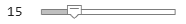15); tic; [tunedParams, bestCost] = tune(tuner,detections,truthTable);```
```Generating code. Code generation successful. Your initial point x0 is not between bounds lb and ub; FMINCON shifted x0 to strictly satisfy the bounds. Iter RMSE Step Size 0 81.1683 0.0000 First-order Norm of Iter F-count f(x) Feasibility optimality step 0 7 8.116826e+01 0.000e+00 2.749e+00 1 14 7.303610e+01 0.000e+00 1.375e+00 2.809e+00 1 73.0361 2.8094 2 21 6.933060e+01 0.000e+00 7.783e-01 3.039e+00 2 69.3306 3.0395 3 28 6.597938e+01 0.000e+00 3.954e-01 4.808e+00 3 65.9794 4.8078 4 35 6.375682e+01 0.000e+00 2.266e-01 5.858e+00 4 63.7568 5.8576 5 42 6.215164e+01 0.000e+00 1.328e-01 7.779e+00 5 62.1516 7.7786 6 49 6.112787e+01 0.000e+00 1.123e-01 9.377e+00 6 61.1279 9.3772 7 56 6.052563e+01 0.000e+00 1.073e-01 1.052e+01 7 60.5256 10.5211 8 63 6.021176e+01 0.000e+00 1.184e-01 9.795e+00 8 60.2118 9.7949 9 70 6.012935e+01 0.000e+00 1.139e-01 2.429e+00 9 60.1293 2.4290 10 77 5.990467e+01 0.000e+00 6.043e-02 7.234e+00 10 59.9047 7.2341 11 84 5.986403e+01 0.000e+00 1.322e-01 6.622e+00 11 59.8640 6.6223 12 91 5.984704e+01 0.000e+00 6.894e-02 4.802e-01 12 59.8470 0.4802 13 98 5.982958e+01 0.000e+00 6.043e-02 2.277e+00 13 59.8296 2.2768 14 105 5.980530e+01 0.000e+00 2.285e-02 5.648e+00 14 59.8053 5.6476 15 112 5.979078e+01 0.000e+00 2.116e-02 7.667e+00 15 59.7908 7.6673 Solver stopped prematurely. fmincon stopped because it exceeded the iteration limit, options.MaxIterations = 1.500000e+01. ```
`disp("Tuning time: " + toc);`
```Tuning time: 74.622 ```
`disp("Best cost: " + bestCost);`
```Best cost: 59.7908 ```
```setTunedProperties(filter,tunedParams); disp("Tuned process noise is:");```
```Tuned process noise is: ```
`disp(filter.ProcessNoise);`
``` 34.9996 50.4336 0 50.4336 83.5728 0 0 0 1.0000 ```
`disp("Tuned initial state covariance is:");`
```Tuned initial state covariance is: ```
`disp(filter.StateCovariance);`
``` 1.0e+04 * 0.9582 0 -0.1154 0 -0.0824 0 0 0.6434 0 0.0231 0 0 -0.1154 0 0.0488 0 -0.3422 0 0 0.0231 0 0.3186 0 0 -0.0824 0 -0.3422 0 4.1085 0 0 0 0 0 0 0.0100 ```
`plotFilterErrors(tuner);`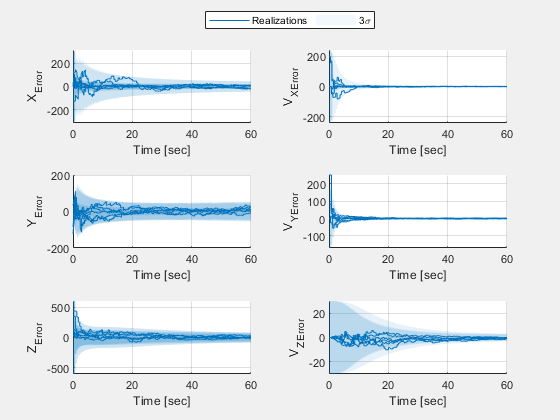Several changes indicate that the result of this tuning is much better than the result of tuning just the process noise. First, the value of the optimal RMSE cost is much lower than it was earlier. Second, the filter errors are all contained inside the 3-sigma bands throughout the scenario time. Finally, these errors and bands get narrower, indicating a good convergence for the filter estimate to the truth value.

### Tune with a Custom Cost

The tuner has two built-in cost metrics: RMSE and normalized estimation error squared (NEES). The RMSE cost strives to minimize the average absolute error but may yield a filter that is either overconfident or underconfident about its estimate. The NEES cost strives to provide a consistent filter based on the cost proposed in . Both RMSE and NEES account for errors in position and, if supplied in the truth table, in velocity.

There are cases where a custom cost allows better tuning. These cases are:

1. When using a truth table that contains a different set of data than position and velocity. Also, the tuner does not validate the truth table when using a custom cost.

2. When using a motion model that is not one of the built-in motion models: `constvelmscjac`, `constaccjac`, `constturnjac`, or `singer`.

3. When the cost must include additional elements or different metrics than the ones defined in the RMSE and NEES metrics.

The `helperCostNormalized` function attached with this example uses the augmented Mahalanobisdistance proposed by Blackman and Popoli . The cost is similar to NEES in the sense that it strives to yield a consistent filter by penalizing both underconfident filter estimates (large state covariance values) and overconfident filter estimates (small state covariance values).

Change the cost metric to the custom metric. You do not need to regenerate code.

```tuner.Cost = "Custom"; tuner.CustomCostFcn = @(trkHistory, truth) helperCostNormalized(trkHistory, truth, "constvel"); tic; [tunedParams, bestCost] = tune(tuner,detections,truthTable);```
```Your initial point x0 is not between bounds lb and ub; FMINCON shifted x0 to strictly satisfy the bounds. Iter Custom Cost Step Size 0 59.7241 0.0000 First-order Norm of Iter F-count f(x) Feasibility optimality step 0 7 5.972415e+01 0.000e+00 3.938e+00 1 14 4.250375e+01 0.000e+00 1.074e+00 5.781e+00 1 42.5038 5.7813 2 21 3.997240e+01 0.000e+00 7.540e-01 2.021e+00 2 39.9724 2.0206 3 28 3.647123e+01 0.000e+00 3.655e-01 4.797e+00 3 36.4712 4.7973 4 35 3.470021e+01 0.000e+00 2.080e-01 4.774e+00 4 34.7002 4.7739 5 42 3.348664e+01 0.000e+00 1.137e-01 5.990e+00 5 33.4866 5.9903 6 49 3.273653e+01 0.000e+00 9.179e-02 6.968e+00 6 32.7365 6.9678 7 56 3.232928e+01 0.000e+00 1.024e-01 6.683e+00 7 32.3293 6.6835 8 63 3.218352e+01 0.000e+00 1.054e-01 3.375e+00 8 32.1835 3.3747 9 70 3.212378e+01 0.000e+00 1.031e-01 1.088e+00 9 32.1238 1.0879 10 77 3.186992e+01 0.000e+00 9.164e-02 6.811e+00 10 31.8699 6.8106 11 84 3.163440e+01 0.000e+00 2.814e-02 1.208e+01 11 31.6344 12.0845 12 91 3.164332e+01 0.000e+00 2.814e-02 7.510e-01 12 31.6433 0.7510 13 98 3.163372e+01 0.000e+00 2.814e-02 8.293e-01 13 31.6337 0.8293 14 105 3.157808e+01 0.000e+00 4.563e-02 4.516e+00 14 31.5781 4.5164 15 112 3.149118e+01 0.000e+00 1.438e-01 8.802e+00 15 31.4912 8.8016 Solver stopped prematurely. fmincon stopped because it exceeded the iteration limit, options.MaxIterations = 1.500000e+01. ```
`disp("The time needed to tune the filter after code generation: " + toc);`
```The time needed to tune the filter after code generation: 58.2451 ```
```setTunedProperties(filter,tunedParams); disp("Tuned process noise is:");```
```Tuned process noise is: ```
`disp(filter.ProcessNoise);`
``` 69.5499 79.3440 0 79.3440 96.3635 0 0 0 1.0000 ```
`disp("Tuned initial state covariance is:");`
```Tuned initial state covariance is: ```
`disp(filter.StateCovariance);`
``` 1.0e+04 * 0.9582 0 -0.1154 0 -0.0824 0 0 0.3330 0 0.0280 0 0 -0.1154 0 0.0488 0 -0.3422 0 0 0.0280 0 0.3875 0 0 -0.0824 0 -0.3422 0 4.1085 0 0 0 0 0 0 0.0100 ```
`plotFilterErrors(tuner);`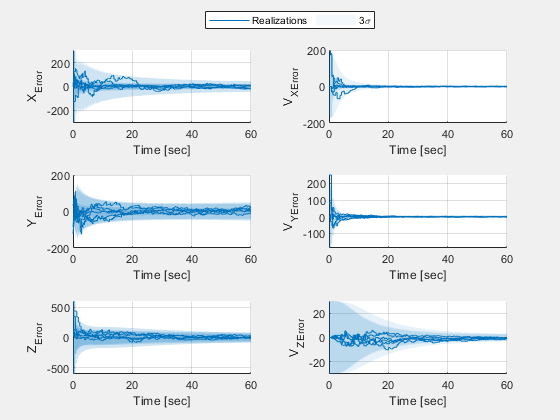`disp("Optimized cost: " + bestCost);`
```Optimized cost: 31.4912 ```

### Use the Tuned Filter in a Tracker

To observe the improvement in tracking quality that a tuned filter provides, use the tuning result in a multitarget tracker. First, use the `exportToFunction` object function of the tuner to export the filter initialization function.

`exportToFunction(tuner, 'myTunedInitFcn');`

Verify the tuned properties of the filter object that is generated by the tuned initialization function.

```filter = myTunedInitFcn(sampleDetection); disp(filter.ProcessNoise);```
``` 69.5499 79.3440 0 79.3440 96.3635 0 0 0 1.0000 ```
`disp(filter.StateCovariance);`
``` 1.0e+04 * 0.9582 0 -0.1154 0 -0.0824 0 0 0.3330 0 0.0280 0 0 -0.1154 0 0.0488 0 -0.3422 0 0 0.0280 0 0.3875 0 0 -0.0824 0 -0.3422 0 4.1085 0 0 0 0 0 0 0.0100 ```

Construct the tracker with this filter initialization function and observe the tracking results. Note that tuning the filter to better handle the initial state uncertainty allow you to reduce the `AssignmentThreshold` property. Having a smaller assignment threshold can help the tracker better assign detections to tracks.

```tracker = trackerGNN(FilterInitializationFcn = "myTunedInitFcn", AssignmentThreshold = 50); helperRunScenario(scenario, tracker, metric);```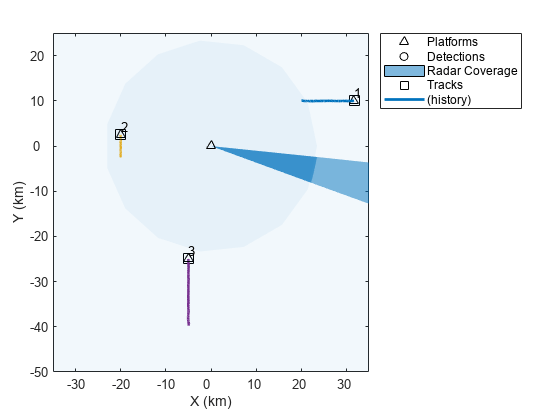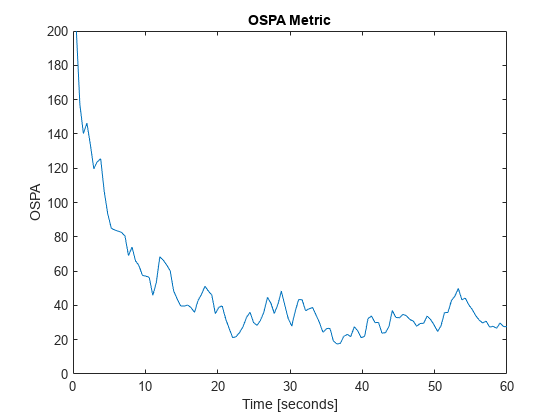The OSPA metric plot above shows a much-improved tracking result. From the metric, once the tracks are established, around 5 seconds into the simulation, the average absolute position error is about 20-80 meters, which is a very good accuracy given the radar measurement accuracy. This result also agrees with the results of the tuning shown in previous sections.

### Summary

In this example, you learned how to automatically tune a tracking filter using a tracking filter tuner. You learned how to control the tuned parameters, how to choose a cost for the tuning, and you used various optimization algorithms. You also learned how to export the tuned filter to a filter initialization function that returns a tuned filter, which can then be used with a multi-target tracker.

### References

 Chen, Z., N. Ahmed, S. Julier, and C. Heckman, “Kalman Filter Tuning with Bayesian Optimization.” ArXiv:1912.08601 [Cs, Eess, Math], Dec. 2019. arXiv.org, http://arxiv.org/abs/1912.08601.

 Blackman, S., and R. Popoli. Design and Analysis of Modern Tracking Systems. Artech House Radar Library, Boston, 1999.

### Supporting Functions

The functions included in this section are intended as helpers for this example and may be removed, modified, or renamed in a future release.

#### helperRecordingToTuningData

Create detection log and truth data from an array of `trackingScenarioRecording` objects.

```function [detlog,truth] = helperRecordingToTuningData(recording) detlog = extractDetections(recording); truth = extractTruth(recording); isemptyDetlog = cellfun(@(dl) isempty(dl), detlog); detlog = detlog(~isemptyDetlog); truth = truth(~isemptyDetlog); end function truth = extractTruth(recording) data = recording.RecordedData; numTruths = numel(data(1).Poses); Time = seconds([data.SimulationTime])'; t = cell(1,numTruths); poses = [data.Poses]; for j = 1:numTruths positions = reshape([poses(j,:).Position],3,[])'; velocities = reshape([poses(j,:).Velocity],3,[])'; t{j} = timetable(Time,positions,velocities,'VariableNames',{'Position','Velocity'}); end truth = repmat(t,1,numel(recording)); end function detlog = extractDetections(recording) numRuns = numel(recording); numTruths = numel(recording(1).RecordedData(1).Poses); detlog = repmat({},1,numTruths * numRuns); logIdx = 0; for runIdx = 1:numRuns data = recording(runIdx).RecordedData; detections = vertcat(data.Detections); for truthIdx = 1:numTruths platID = data(1).Poses(truthIdx).PlatformID; fromThisPlat = cellfun(@(d) d.ObjectAttributes{1}.TargetIndex == platID, detections); logIdx = logIdx + 1; detlog{logIdx} = detections(fromThisPlat); end end end```

#### helperVisualizeTuningData

This function visualizes the tuning data and returns the `theaterPlot` visualization object.

```function helperVisualizeTuningData(detections, truthTable) thp = theaterPlot(AxesUnits=["km","km","km"],XLimits=[-35000 35000],YLimits=[-50000 25000]); trp = trajectoryPlotter(thp,DisplayName="Truth Trajectories"); numTruths = numel(truthTable); pos = cell(1,numTruths); for i = 1:numTruths pos{i} = truthTable{i}.Position; end plotTrajectory(trp,pos); knownPlatforms = []; existingDetPlotters = {}; colors = colororder; for j = 1:numTruths d = vertcat(detections{j}{:}); if ~isempty(d) platIndex = d(1).ObjectAttributes{1}.TargetIndex; if ~any(platIndex == knownPlatforms) dtp = detectionPlotter(thp,DisplayName=("Platform"+platIndex+"Detections"),MarkerEdgeColor=colors(platIndex,:)); knownPlatforms(end+1) = platIndex; %#ok<*AGROW> existingDetPlotters{end+1} = dtp; else dtp = existingDetPlotters{platIndex == knownPlatforms}; end detpos = arrayfun(@(d) d.Measurement, d, UniformOutput=false); plotDetection(dtp,[detpos{:}]'); end end end```

#### helperRunScenario

A function to run a recording, track the objects, visualize the results, and analyze tracking quality.

```function helperRunScenario(scenario, tracker, metric) % Initialize plotters persistent thp plp dep cvp tap if isempty(thp) thp = theaterPlot(AxesUnits=["km","km","km"],XLimits=[-35000 35000],YLimits=[-50000 25000]); end if isempty(plp) plp = platformPlotter(thp,DisplayName="Platforms"); end if isempty(dep) dep = detectionPlotter(thp,DisplayName="Detections"); end if isempty(cvp) cvp = coveragePlotter(thp,DisplayName="Radar Coverage"); end if isempty(tap) tap = trackPlotter(thp, DisplayName="Tracks", ConnectHistory="on", ColorizeHistory="on"); end % Reset all objects clearPlotterData(thp); restart(scenario); reset(tracker); reset(metric); % Modify the radar to rotate faster and provide more detections radar = scenario.Platforms{1}.Sensors{1}; release(radar); radar.FieldOfView(1) = 14; radar.MaxMechanicalScanRate(1) = 750; % Initialize variables. There are 3215 timestamps in this scenario ospa = zeros(1,3215); trackerTimes = zeros(1,3215); detBuffer = {}; tracks = objectTrack.empty; index = 0; % For repeatable results, use a constant RNG seed r = rng(0,'twister'); h = onCleanup(@() rng(r)); while advance(scenario) time = scenario.SimulationTime; poses = platformPoses(scenario); covcon = coverageConfig(scenario); [detections, sencon] = detect(scenario); if ~sencon.IsScanDone detBuffer = vertcat(detBuffer,detections); else tracks = tracker(detBuffer, time); index = index + 1; ospa(index) = metric(tracks,poses(2:4)); trackerTimes(index) = time; detBuffer = {}; end if isempty(tracks) trkpos = zeros(0,3); trkIDs = string.empty; else trkpos = getTrackPositions(tracks,"constvel"); trkIDs = string([tracks.TrackID]); end if isempty(detBuffer) detpos = zeros(0,3); else detpos = cellfun(@(db) db.Measurement, detBuffer, 'UniformOutput', false); detpos = [detpos{:}]'; end plotPlatform(plp, reshape([poses.Position],3,[])'); plotCoverage(cvp, covcon); plotDetection(dep, detpos); plotTrack(tap, trkpos, trkIDs); end figure; plot(trackerTimes(1:index),ospa(1:index)); ylim([0 200]); title('OSPA Metric'); xlabel('Time [seconds]'); ylabel('OSPA'); end```

#### helperCostNormalized

Calculate the Blackman and Popoli augmented Mahalanobis distance.

Given the error between the state at time step k and Monte Carlo run i and the state estimate at that time, ${\mathit{x}}_{\mathrm{err}}\left(\mathit{k},\mathit{i}\right)=\mathit{x}\left(\mathit{k},\mathit{i}\right)-{\mathit{x}}_{\mathrm{est}}\left(\mathit{k},\mathit{i}\right)$, with a state covariance P(k,i), the Blackman and Popoli cost is:

`$\mathit{d}\left(\mathit{k},\mathit{i}\right)={\mathit{x}}_{\mathrm{err}}{\left(\mathit{k},\mathit{i}\right)}^{\prime }\text{\hspace{0.17em}}{\mathit{P}\left(\mathit{k},\mathit{i}\right)}^{-1}{\mathit{x}}_{\mathrm{err}}\left(\mathit{k},\mathit{i}\right)\text{\hspace{0.17em}}+\text{\hspace{0.17em}}\mathrm{log}\left(\mathrm{det}\left(\mathit{P}\left(\mathit{k},\mathit{i}\right)\right)\right)\text{\hspace{0.17em}\hspace{0.17em}\hspace{0.17em}\hspace{0.17em}\hspace{0.17em}\hspace{0.17em}\hspace{0.17em}\hspace{0.17em}\hspace{0.17em}\hspace{0.17em}\hspace{0.17em}\hspace{0.17em}\hspace{0.17em}\hspace{0.17em}\hspace{0.17em}\hspace{0.17em}\hspace{0.17em}\hspace{0.17em}\hspace{0.17em}\hspace{0.17em}\hspace{0.17em}\hspace{0.17em}\hspace{0.17em}\hspace{0.17em}\hspace{0.17em}}\forall \mathit{k}\in 1,...,\mathit{T},\forall \text{\hspace{0.17em}}\mathit{i}\in 1,...,\mathit{N}$`

T is the number of timesteps. N is the number of Monte Carlo runs.

Note that the $\mathrm{log}\left(\mathrm{det}\left(\mathit{P}\left(\mathit{k},\mathit{i}\right)\right)\right)$ term is added to penalize large values of state covariance whereas the Mahalanobis cost term serves to penalize overconfident filter estimates.

The overall cost is the average of $\mathit{d}\left(\mathit{k},\mathit{i}\right)$ over all timesteps and runs.

```function cost = helperCostNormalized(trkHistory, truthTable, model) % Process truthTable to position and velocity truths posTruth = [truthTable.Position]; hasVelocity = ismember('Velocity', truthTable.Properties.VariableNames); if hasVelocity velTruth = [truthTable.Velocity]; end [numSteps, numRuns] = size(trkHistory); c = zeros(numSteps, numRuns, 'like', posTruth); for run = 1:numRuns [posEst, posCovs] = getTrackPositions(trkHistory(:,run),model); [velEst, velCovs] = getTrackVelocities(trkHistory(:,run),model); if hasVelocity poserror = posTruth - posEst; velerror = velTruth - velEst; stateerror = [poserror,velerror]; else stateerror = posTruth - posEst; end for step = 1:numSteps if hasVelocity P = blkdiag(posCovs(:,:,step), velCovs(:,:,step)); else P = posCovs(:,:,step); end c(step,run) = stateerror(step,:) /P * stateerror(step,:)' + log(det(P)); end end cost = mean(c,"all"); end```